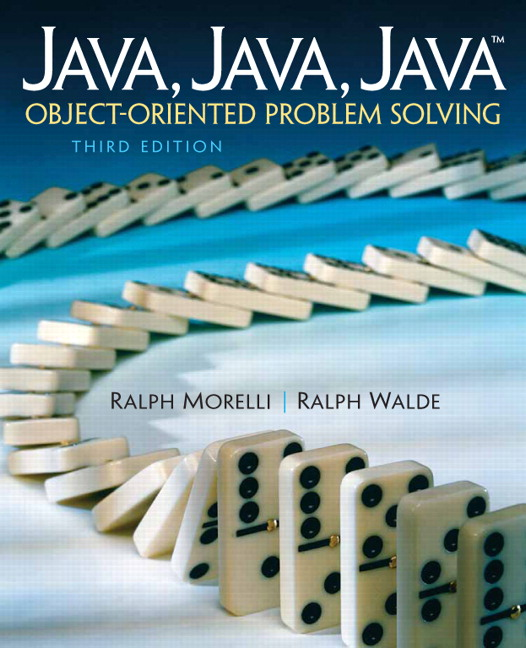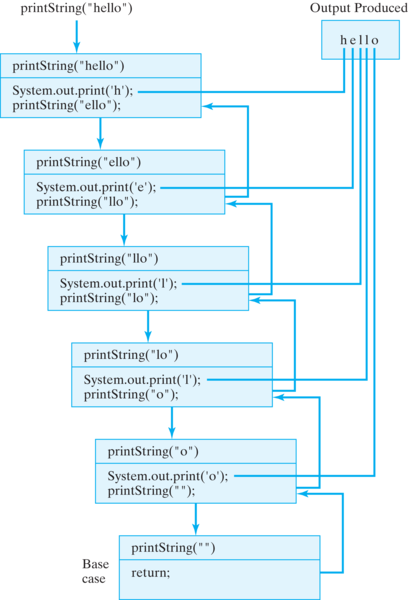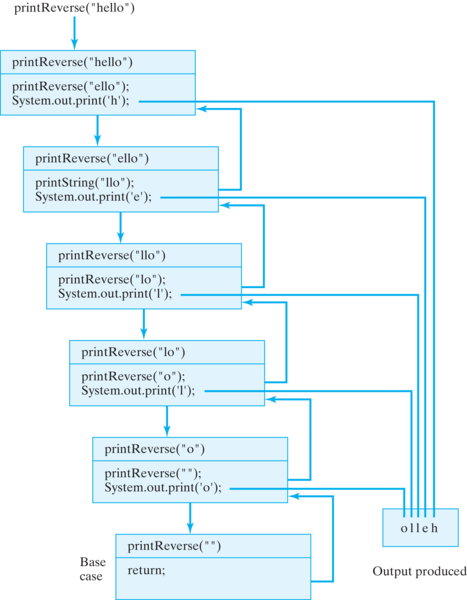# Java, Java, Java: Object-Oriented Problem Solving, 2022E

## Section12.3Recursive String Methods

Remember that a recursive method is a method that calls itself. Like recursive definitions, recursive methods are designed around the divide-and-conquer and self-similarity principles. Defining a recursive method involves a similar analysis to the one we used in designing recursive definitions. We identify a self-similar subproblem of the original problem plus one or more limiting cases.
The idea of a method calling itself seems a bit strange at first. It’s perhaps best understood in terms of a clone or a copy. When a method calls itself, it really calls a copy of itself, one that has a slightly different internal state. Usually the difference in state is the result of a difference in the invoked method’s parameters.

### Subsection12.3.1Printing a String

To illustrate the concept of a recursive method, let’s define a recursive method for printing a string. This is not intended to be a practical method—we already have the println() method for printing strings. But pretend for a moment that you only have a version of println() that works for characters, and your task is to write a version that can be used to print an entire string of characters.
A little terminology will help us describe the algorithm. Let’s call the first letter of a string the head of the string, and let’s refer to all the remaining letters in the string as the tail of the string. Then the problem of printing a string can be solved using a head-and-tail algorithm, which consists of two parts: printing the head of the string and recursively printing its tail. The limiting case here is when a string has no characters in it. It’s trivial to print the empty string—there is nothing to print! This leads to the method definition shown in Listing 12.3.1.
The base case here provides a limit and bounds the recursion when the length of s is 0—that is, when the string is empty. The recursive case solves the problem of printing s by solving the smaller, self-similar problem of printing a substring of s. Note that the recursive case makes progress toward the limit. On each recursion, the tail will get smaller and smaller until it becomes the empty string.
Let’s now revisit the notion of a method calling itself. Obviously this is what happens in the recursive case, but what does it mean—what actions does this lead to in the program?
Each recursive call to a method is really a call to a copy of that method, and each copy has a slightly different internal state. We can define printString()’s internal state completely in terms of its recursion parameter, s, which is the string that’s being printed. A recursion parameter is a parameter whose value is used to control the progress of the recursion. In this case, if s differs in each copy, then so will s.substring(1) and s.charAt(0).
Figure 12.3.4 illustrates the sequence of recursive method calls and the output that results when printString("hello") is invoked.Figure 12.3.4. A recursive method call invokes a copy of the method, each with a slightly different internal state. As this is done repeatedly, a stack of method calls is created.
Each box represents a separate instance of the printString() method, with its own internal state. In this illustration, its state is represented by its parameter, s. Because each instance has a different parameter, behaviors differ slightly. Each box shows the character that will be printed by that instance (s.charAt(0)), and the string that will be passed on to the next instance (s.substring(1)).
The arrows represent the method calls and returns. Note that the first return executed is the one in the base case. Each instance of the method must wait for the instance it called to return before it can return. That’s why the instances “pile up” in a cascade-like structure. The arrowless lines trace the order in which the output is produced.
Each instance of printString() is similar to the next in that each will print one character and then pass on a substring, but each performs its duties on a different string. Note how the string, the recursion parameter in this case, gets smaller in each instance of printString(). This represents progress toward the method’s base case s.length() == 0. When the empty string is passed as an argument, the recursion will stop. If the method does not make progress toward its bound in this way, the result will be an infinite recursion.
Note also the order in which things are done in this method. First s.charAt(0) is printed, and then s.substring(1) is passed to printString() in the recursion. This is a typical structure for a head-and-tail algorithm. What makes this work is that the tail is a smaller, self-similar version of the original structure.

#### ExercisesSelf-Study Exercise

##### 1.Tracing printString().
Use Codelens to step through the printString() method, taking care to notice the different state of the method on each recursive call. It should match Figure 12.3.4.
##### 2.What’s the output?
What would be printed by the following version of the printString2() method, if it is called with printString2("hello")?
public void printString2(String s)  {
if (s.length() == 1)
System.out.print(s.charAt(0));         // Base case:
else {                                   // Recursive case
System.out.print(s.charAt(s.length() - 1));
printString2(s.substring(0, s.length() - 1));
}
} // printString2()

##### 3.Tracing printString2().
Use Codelens to step through the printString2() method, taking care to notice the different state of the method on each recursive call.

### Subsection12.3.2Printing the String Backward

What do you suppose would happen if we reversed the order of the statements in the printString() method? That is, what if the recursive call came before s.charAt(0) is printed, as in the following method:
/**
* printReverse() prints each character s in reverse order
* Pre: s is initialized (non-null)
* Post: none
*/
public void printReverse(String s) {
if (s.length() > 0) {      // Recursive case:
printReverse(s.substring(1));  // Print tail
System.out.print(s.charAt(0)); // Print first char
}
} // printReverse()

As its name suggests, this method will print the string in reverse order. The trace in Figure 12.3.8 shows how this works.Figure 12.3.8. A trace of printReverse(s), which prints its string argument in reverse order.
Before printReverse("hello") can print h, it calls printReverse("ello") and must wait for that call to complete its execution and return. But printReverse("ello") calls printReverse("llo") and so must wait for that call to complete its execution and return.
This process continues until printReverse("") is called. While the base case is executing, the other five instances of printReverse() must each wait for the instance that they called to complete its execution. It is only after the base case returns, that printReverse("o") can print its first character and return.
So the letter o will be printed first. After printReverse("o") has returned, then printReverse("lo") can print its first character. So the letter l will be printed next, and so on, until the original call to printReverse("hello") is completed and returns. Thus, the string will be printed in reverse order.
Note that the method call and return structure in this example follows a last-in-first-out (LIFO) protocol. That is, the last method called is always the first method to return. This is the protocol used by all method calls, recursive or otherwise.
For example, compare the order in which things happen in Figure 12.3.8 with the method stack trace in Figure 12.3.4. The only real difference between the two figures is that here the method stack is represented as growing downward, whereas in Figure 12.3.4 it grows upward. As each method call is made, a representation of the method call is placed on the method call stack. When a method returns, its block is removed from the top of the stack. The only difference between recursive and nonrecursive method calls is that recursive methods call instances of the same method definition. Of course, as we’ve seen, the instances are all slightly different from each other.

#### ExercisesSelf-Study Exercises

##### Activity12.3.1.Tracing printReverse().
Use Codelens to step through the printReverse() method, taking care to notice the different state of the method on each recursive call. It should match Figure 12.3.8.
##### 1.Count down.
Write a recursive method called countDown() that takes a single int parameter, $$N \geq 0\text{,}$$ and prints a countdown, such as “5, 4, 3, 2, 1, blastoff.” The main program is completed for you.
##### 2.Count down by two.
Revise the method in the previous exercise so that when it’s called with countDown(10), it will print “10 8 6 4 2 blastoff”; if it’s called with countDown(9), it prints “9 7 5 3 1 blastoff.” The main program is completed for you.

### Subsection12.3.3Counting Characters in a String

Suppose you’re writing an encryption program and you need to count the frequencies of the letters of the alphabet. Let’s write a recursive method for this task.
This method will have two parameters: a String to store the string that will be processed and a char to store the target character—the one we want to count. The method should return an int, representing the number of occurrences of the target character in the string:
// Goal: count the occurrences of ch in s
public int countChar(String s, char ch) {
...
}

Here again our analysis must identify a recursive step that breaks the problem into smaller, self-similar versions of itself, plus a base case or limiting case that defines the end of the recursive process. Because the empty string will contain no target characters, we can use it as our base case. So, if it is passed the empty string, countChar() should just return 0 as its result.
For the recursive case, we can divide the string into its head and tail. If the head is the target character, then the number of occurrences in the string is (1 + the number of occurrences in its tail). If the head of the string is not the target character, then the number of occurrences is (0 + the number of occurrences in its tail). Of course, we’ll use recursion to calculate the number of occurrences in the tail.
This analysis leads to the recursive method shown in Listing 12.3.10. Note that for both recursive cases the same recursive call is used.
In both cases we pass the tail of the original string, plus the target character. Note also how the return statement is evaluated:
return 1 + countChar(s.substring(1),ch); // Head = ch

Before the method can return a value, it must receive the result of calling countChar(s.substring(1),ch) and add it to 1. Only then can a result be returned to the calling method. This leads to the following evaluation sequence for countChar("dad",'d'):
countChar("dad",'d');
1 + 0 + countChar("d",'d');
1 + 0 + 1 + countChar("",'d');
1 + 0 + 1 + 0 = 2              // Final result

In this way, the final result of calling countChar("dad",'d') is built up recursively by adding together the partial results from each separate instance of countChar(). The evaluation process is shown graphically in Figure 12.3.11.

#### ExercisesSelf-Study Exercises

##### Activity12.3.2.Tracing countChar().
Use Codelens to step through the countChar() method, taking care to notice the different state of the method on each recursive call. It should match Figure 12.3.11.
##### 1.Sum of 1 to N.
Here’s a numerical problem. Write a recursive method to compute the sum of 1 to N, given N as a parameter. That is, $$sum1toN(N) = 1+2+3+...+N\text{,}$$ for $$N >= 0\text{.}$$

### Subsection12.3.4Translating a String

A widely used string-processing task is to convert one string into another string by replacing one character with a substitute throughout the string. For example, suppose we want to convert a Unix path name, which uses the forward slash “/” to separate one part of the path from another, into a Windows path name, which uses the backslash character “$$\backslash$$” as a separator. For example, we want a method that can translate the following two strings into one another:
/unix_system/myfolder/java
\Windows_system\myfolder\java

Thus, we want a method that takes three parameters: a String, on which the conversion will be performed, and two char variables, the first being the original character in the string and the second being its substitute. The precondition for this method is simply that each of these three parameters has been properly initialized with a value. The postcondition is that all occurrences of the first character have been replaced by the second character.
As in our previous string-processing methods, the limiting case in this problem is the empty string, and the recursive case will divide the string into its head and its tail. If the head is the character we want to replace, we concatenate its substitute with the result we obtain by recursively converting its tail.
This analysis leads to the definition shown in Listing 12.3.13. This method has more or less the same head and tail structure as the preceding example. The difference is that here the operation we perform on the head of the string is concatenation rather than addition.
The base case is still the case in which str is the empty string. The first recursive case occurs when the character being replaced is the head of str. In that case, its substitute (ch2) is concatenated with the result of converting the rest of the string and returned as the result. The second recursive case occurs when the head of the string is not the character being replaced. In this case, the head of the string is simply concatenated with the result of converting the rest of the string. Figure 12.3.14 shows an example of its execution.

#### ExercisesSelf-Study Exercise

##### Activity12.3.3.Tracing convert().
Use Codelens to step through the convert() method, taking care to notice the different state of the method on each recursive call. It should match Figure 12.3.14.
Write a recursive method,  addBlanks() that changes each blank in a string into two consecutive blanks, leaving the rest of the string unchanged.

### Subsection12.3.5Printing all Possible Outcomes when Tossing N Coins

Suppose that a student who is studying probability wishes to have a Java program that, for any positive integer $$N\text{,}$$ will print out a list of all possible outcomes when $$N$$ coins are tossed. For purposes of analyzing the problem, it is assumed that the coins are numbered $$1$$ through $$N$$ and that they are tossed one at a time. An outcome will be represented by a string of H’s and T’s corresponding to heads and tails.
Thus, if $$N = 2\text{,}$$ the string HT represents a head on the first coin and a tail on the second coin. What we need is a method which, when given the number of coins, will print out all strings of this type. In case of two coins the output should be:
HH
HT
TH
TT

Let’s devise a strategy, given any positive integer $$N\text{,}$$ for printing the strings that correspond to all possible outcomes when tossing $$N$$ coins. Clearly, for $$N = 1\text{,}$$ the method needs to print an H on one line and a T on the next line.
For an arbitrary number of coins $$N\text{,}$$ one way to generate all outcomes is to think of two kinds of strings—those that start with an H and those that start with a T. The strings that start with H can be generated by inserting an H in front of each of the outcomes that occur when $$N-1$$ coins are tossed. The strings beginning with T can be generated in a similar manner. Thus, using the outcomes for two coins above, we know that the outcomes for three coins for which the first coin is H are:
HHH
HHT
HTH
HTT

Using an argument similar to the one above, we can generalize this to a description of the recursive case for an algorithm. We want an algorithm that generates all those outcomes for $$N$$ coins where we are given a string STR representing one particular outcome for all but the last $$K$$ coins where $$0 \lt K \lt = N\text{.}$$
To print out all such outcomes, just print all outcomes with a fixed outcome corresponding to STR + "H" for all but the last $$K - 1$$ coins and then print all outcomes with the fixed outcome STR + "T" for all but the last $$K - 1$$ coins. The base case is the special case $$K = 1$$ when you just need STR + "H" on one line and STR + "T" on the next. If you start the algorithm with STR = "" and $$K = N\text{,}$$ this algorithm will print out all the outcomes for tossing $$N$$ coins.
To translate this into Java code we can create a method called printOutcomes(String str,int N). The above recursive description easily translates into code for the method in Listing 12.3.15.
To print out all outcomes when tossing, say, seven coins, just make the method call CoinToss.printOutcomes("",7). This particular call would generate the desired output:
HHHHHHH
HHHHHHT
.......
TTTTTTH
TTTTTTT

To better understand how the recursive method definition generates its output, it might be helpful to trace the order of recursive calls and output to System.out that occurs when executing printOutcomes("",3) as shown in Figure 12.3.16.
Use Codelens to step through the printOutcomes> method, taking care to notice the different state of the method on each recursive call. Note that in this algorithm there are two recursive calls in the recursive case. It should match Figure 12.3.16.
Modify the above printOutcomes() method so that it will print out all possible outcomes when a chess player plays a series of $$N$$ games. The outcome of each game is to be represented by a W, L, or D corresponding to the player winning, losing, or drawing the game.